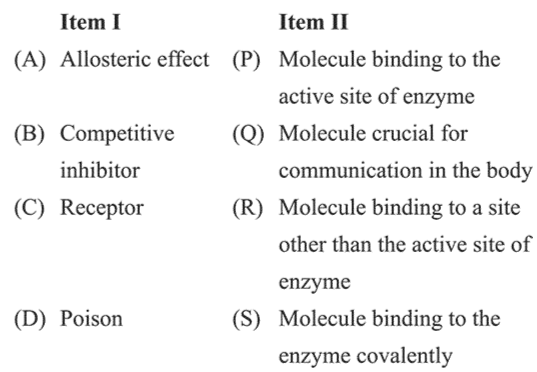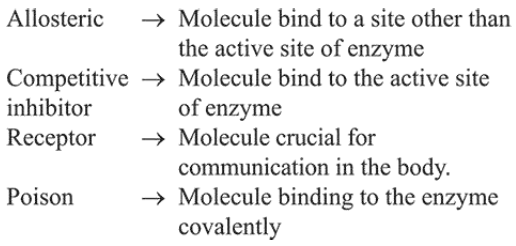# The correct match between Item I and Item II is:Question:

The correct match between Item I and Item II is:1. $(\mathrm{A}) \rightarrow(\mathrm{R}) ;(\mathrm{B}) \rightarrow(\mathrm{P}) ;(\mathrm{C}) \rightarrow(\mathrm{Q}) ;(\mathrm{D}) \rightarrow(\mathrm{S})$

2. $(\mathrm{A}) \rightarrow(\mathrm{P}) ;(\mathrm{B}) \rightarrow(\mathrm{R}) ;(\mathrm{C}) \rightarrow(\mathrm{Q}) ;(\mathrm{D}) \rightarrow(\mathrm{S})$

3. $(\mathrm{A}) \rightarrow(\mathrm{R}) ;(\mathrm{B}) \rightarrow(\mathrm{P}) ;(\mathrm{C}) \rightarrow(\mathrm{S}) ;(\mathrm{D}) \rightarrow(\mathrm{Q})$

4. $(\mathrm{A}) \rightarrow(\mathrm{P}) ;(\mathrm{B}) \rightarrow(\mathrm{R}) ;(\mathrm{C}) \rightarrow(\mathrm{S}) ;(\mathrm{D}) \rightarrow(\mathrm{Q})$

Correct Option: 1

Solution: